• # 定义一个链表 class Node: """ 定义节点类 data:数据 _next:下一个数据 """ def __init__(self, data, _next=None): self.data = data self._next = _next def __repr__(self): """ 用来定义Node的...
# 定义一个链表
class Node:
"""
定义节点类
data:数据
_next:下一个数据
"""

def __init__(self, data, _next=None):
self.data = data
self._next = _next

def __repr__(self):
"""
用来定义Node的字节输出, __repr__()方法是object类提供的方法，
所有的Python对象都具有__repr__() 方法。它是一个“自我描述”的方法，

该方法通常实现场景是：当开发人员直接打印该对象时，
系统将会输出该对象的“自我描述”信息，用来告诉外界该对象具有的状态信息。

object类提供的__repr__()方法总是返回该对象实现类的“类名+object at + 内存地址 ”值，
这个返回值并不能真正实现“自我描述”的功能，
因此如果用户需要自定义类能实现“自我描述”的功能，就必须重写__repr__()方法。
"""
return str(self.data)

class ChainTable(Node):
"""
定义链表
"""
def __init__(self):
self.length = 0

def isEmpty(self):
return (self.length == 0)

item = None
if isinstance(dataOrNode, Node):
item = dataOrNode
else:
item = Node(dataOrNode)

self.length += 1
else:
while node._next:  # 当有节点时，新增下一个节点
node = node._next
node._next = item
self.length += 1

def delete(self, index):
if self.isEmpty():
print("链表是空的")
return
if index < 0 or index >= self.length:
print("超出索引长度")
return

# 删除第一个节点
if index == 0:
self.length -= 1
return

# prev为保存前导节点
# node为保存当前节点
# 当j与index相等时就
# 相当于找到要删除的节点
j = 0
while node._next and j < index:
prev = node
node = node._next
j += 1
if j == index:  # 找到节点后将下一个节点覆盖到本节点
prev._next = node._next
self.length -= 1

def update(self, index, data):
if self.isEmpty() or index < 0 or index >= self.length:
print('索引超出范围')
return
j = 0
while node._next and j < index:
node = node._next
j += 1
if j == index:
node.data = data

# 查找一个节点
def getItem(self, index):
if self.isEmpty() or index < 0 or index >= self.length:
print('超出索引长度')
return
j = 0
while node._next and j < index:
node = node._next
j += 1
return node.data

# 从头到尾打印链表
def print_chain(self):
if self.isEmpty():
print('链表为空')
num = []
while node:
num.append(node)
node = node._next
return num

# 查找一个节点的索引
def getIndex(self, data):
j = 0
if self.isEmpty():
print('链表为空')
return
while node:
if node.data == data:
return j
node = node._next
j += 1
if j == self.length:
return

def insert(self, index, dataOrNode):
if self.isEmpty():
print('链表为空')
return
if index < 0 or index >= self.length:
print('超出索引长度')
return
item = None
if isinstance(dataOrNode, Node):
item = dataOrNode
else:
item = Node(dataOrNode)
if index == 0:
self.length += 1
return
j = 0
while node._next and j < index:
prev = node
node = node._next
j += 1
if j == index:
item._next = node
prev._next = item
self.length += 1

def clear(self):
self.length = 0

def __repr__(self):
if self.isEmpty():
return "链表为空"
nlist = ''
while node:
nlist += str(node.data) + ' '
node = node._next
return nlist

def __getitem__(self, ind):
if self.isEmpty() or ind < 0 or ind >= self.length:
print("超出索引长度")
return
return self.getItem(ind)

def __setitem__(self, ind, val):
if self.isEmpty() or ind < 0 or ind >= self.length:
print("超出索引长度")
return
self.update(ind, val)

def __len__(self):
return self.length

chainTable = ChainTable()
for i in range(10):
print(chainTable.getIndex(1))
print(chainTable.print_chain())

在节点的删除操作，和对链表的销毁操作中。不必担心太多节点储存在内存中而出现内存泄漏的现象。因为Python有自动垃圾回收机制。当然如果觉得不删除节点不爽的话，可以调用对象的del()方法。
参考文章
展开全文python 数据结构
• ## C语言创建链表

千次阅读 2019-10-23 13:05:32
C语言创建链表 一、链表中结点的存储        链表的结点左边一部分是存放的数据，右边一部分是后继指针指向下一个结点的地址。C语言中通常定义一个结构体类型来存储一个结点，如下： struct ...


C语言创建链表

一、链表中结点的存储
链表的结点左边一部分是存放的数据，右边一部分是后继指针指向下一个结点的地址。C语言中通常定义一个结构体类型来存储一个结点，如下：

struct node
{
int data;
struce node *next; //下一个结点的类型也是struct node，所以后继指针的类型也必须是struct node *
};

二、让我们把结点连起来吧
struct node *head;

现在我们来创建第一个结点，并用临时指针p指向这个结点。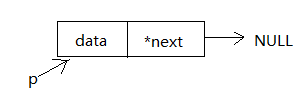struct node *p;
p=(struct node *)malloc(sizeof(struct node));  //为新结点动态申请一个空间，并用临时结点p指向这个新地址
scanf("%d",&a);  //读取数据
p->data=a; //将数据存储到当前结点的data域中
p->next=NULL;  //设置当前结点的后继指针指向空，也就是当前结点的下一个结点为空

把新加入的结点串进链表。如果该结点是创建的第一个结点，则将头指针指向这个结点再将当前指针指向这个结点；如果该结点不是第一个，则将上一个结点的后继指针指向该结点再修改当前指针指向这个新结点。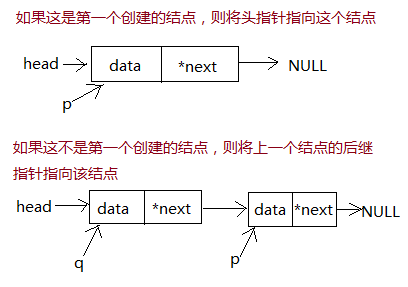if(head==NULL)
else
q->next=p;
q=p; //最后指针q也指向当前结点

三、建立链表并遍历输出的完整代码
#include <stdio.h>
#include <stdlib.h>
struct node
{
int data;
struct node *next;
};
int main()
{
int i,n,a;
scanf("%d",&n);
for(i=0;i<n;i++){
p=(struct node *)malloc(sizeof(struct node));
scanf("%d",&a);
p->data=a;
p->next=NULL;
else
q->next=p;
q=p;
}
//输出链表
while(t!=NULL){
printf("%d ",t->data);
t=t->next;
}
return 0;
}

展开全文• 链表就是很多个结构体通过地址相关联(至少我目前了解到的是这样)，每个结构体里由两部分：一是数据，二是下一个结构体的地址，于是就可以通过一个结构体获取下一个结构的地址，然后通过这个地址访问它的数据，再获取...
链表就是很多个结构体通过地址相关联(至少我目前了解到的是这样)，每个结构体里由两部分：一是数据，二是下一个结构体的地址，于是就可以通过一个结构体获取下一个结构的地址，然后通过这个地址访问它的数据，再获取下一个地址，往复。形成一种链状数据结构，如图：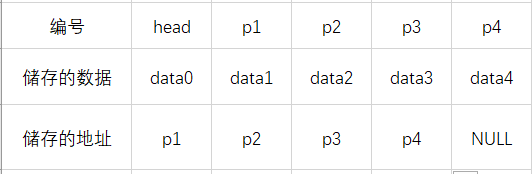1. 先看我最先接触到的一种写法(无头&&尾插法)，看题：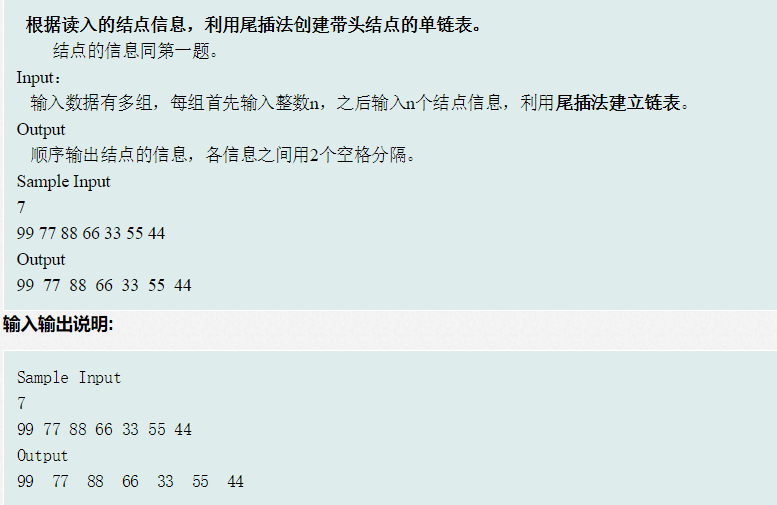#include <stdio.h>
#include <stdlib.h>
#include <string.h>
struct node{                //节点结构体
int data;
struct node *next; //记录下一节点地址
};
int main()
{
int n,i;
while(scanf("%d",&n)!=-1)
{
for(i=0;i<n;i++)        //建立n个节点的链表
{

p=(struct node *)(malloc(sizeof(struct node))); //动态申请空间得到空间地址，并将之强
//制类型转换为节点结构体类型指针，赋给p

scanf("%d",&p->data);    //其实是&(p->data)，这里不能再用"."，因为p是地址

else                     //后面的操作就是把上一节点的next赋值为当前p(第一次先跳过)
q->next=p;

p->next=NULL;            //把当前指针的next赋值为空，其实是为尾部next为空做贡献
q=p;                     //记录一下本次循环得到的指针，p马上要被覆盖

}

while(p!=NULL)        //可以直接while(p)
{
if(i==n-1) printf("%d\n",p->data);
else printf("%d  ",p->data);
p=p->next;
}

}
}



如果还不是不能理解，跟着循环自己画一下链状图应该就差不多了，逻辑和我给出的第一张图一样。
2. 有头&&头插法(感觉头插法不是很好理解，最好先分析一下代码，画张图，否则可能还是不懂，画完再看注释)：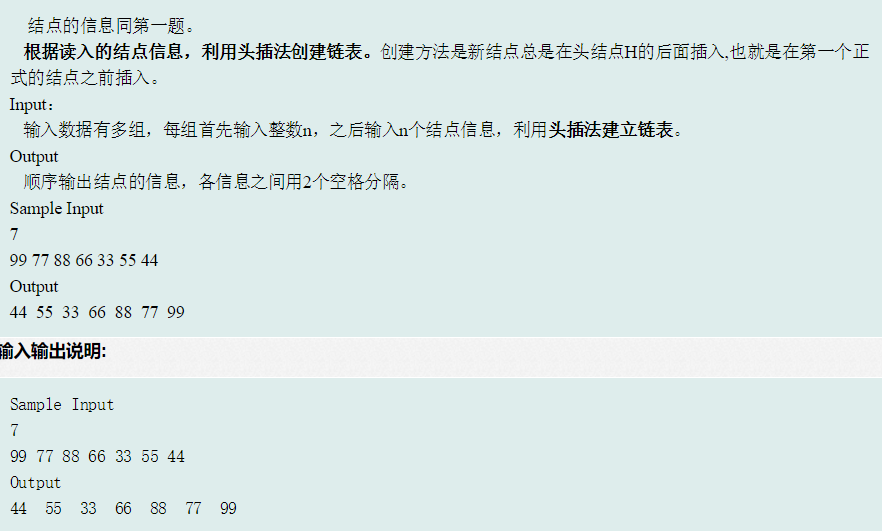看代码：
#include <stdio.h>
#include <stdlib.h>
#include <string.h>
struct node{
int data;
struct node *next;
};
int main()
{
int n,i;
while(scanf("%d",&n)!=-1)
{
for(i=0;i<n;i++)
{
p=(struct node *)(malloc(sizeof(struct node)));    //申请新节点
scanf("%d",&p->data);        //节点赋数据
//如果理解我的意思的话不用背也应该知道该怎么写了
}

while(p!=NULL)
{
if(i==n-1) printf("%d\n",p->data);
else printf("%d  ",p->data);
p=p->next;
}
}
}

3. 链表的基本操作：
输出：上面一直在用，就不说了。
增加：记录当前指针p和上一次指针q，找到位置时q->next=t; t->next=p;即可，但注意有头与无头时头部和尾部插入的处理。
删除：记录当前指针p和上一次指针q，找到时q->next=p->next即可，注意有头和无头时删除头节点的处理。
修改：记录当前指针p，找到修改即可。
查询：记录当前指针p，判断是否符合条件。
排序：
//1. 冒泡排序，交换链表单元上的值

while(p)
{
q=p->next;
while(q)
{
if(q->num<p->num)
{
t=q->num;
q->num=p->num;
p->num=t;
}
q=q->next;
}
p=p->next;
}

//2. 有序插入

//本题要求降序排列

for(i=0;i<n;i++)
{
p=(struct stu *)malloc(sizeof(struct stu));
scanf("%u %s %lf %lf %lf",&p->num,p->name,&p->classa,&p->classb,&p->classc);
p->sum=(p->classa+p->classb+p->classc);
p->aver=(p->sum)/3.0;
if(i==0)
{
p->next=NULL;
q=p;
}
else
{
while(tp!=NULL && (tp->sum)>(p->sum))    //找到第一个小于p的位置
{
tq=tp;
tp=tp->next;
}
p->next=t;
q=t;
}else if(tp==NULL){
q->next=p;
p->next=NULL;
q=p;
}else{
t=tq->next;
tq->next=p;
p->next=t;
}
}
}


逆序：
方法一：新建链表，用头插法将原表数据复制到新链表。
方法二：对原链表进行操作，比较难理解，自己最好画图看一下。
#include <stdio.h>
#include <stdlib.h>
struct NUM{
int data;
struct NUM *next;
};
int main()
{
int n,t,i;                                //用尾插法创建有头链表1-
while(scanf("%d",&n)!=-1)
{
for(i=0;i<n;i++)
{
scanf("%d",&t);
p=(struct NUM *)malloc(sizeof(struct NUM));
p->data=t;
else q->next=p;
p->next=NULL;
q=p;
}                                      //链表创建结束-1

while(p->next)
{
q=p->next;                         //取当前节点的下一个节点
p->next=q->next;                   //当前节点直接连接到下一个节点的下一个节点

}                                       //链表逆序-2

while(p)
{
printf("%d  ",p->data);
p=p->next;
}

}
}

不大明白的话可以看下图：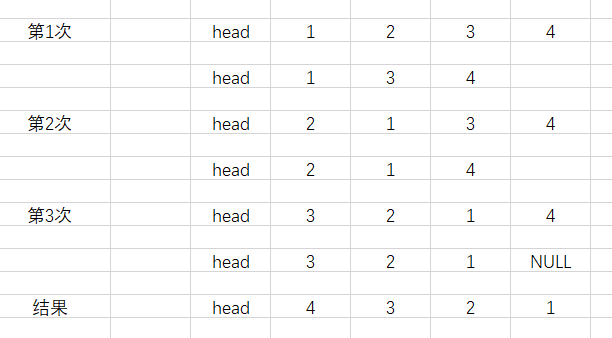如果有问题的话，欢迎留言。
展开全文链表操作 链表排序 链表逆序 c语言
1。尾插法
工作原理：
代码实现：
import java.util.Random;

int data;    //创建一个数组的属性
Random r = new  Random();//随机对象
//storage 为 永远存储最后一个节点的地址
//last 为  新的临时节点
head = storage = last = null;
for (int i = 0; i <len ; i++) {
int temp = r.nextInt(50);
last.data = temp;  //为属性赋值
}else {
storage.next = last;//将新节点连接到链表的尾部
storage = last;  //永远的储存最后一个节点的地址
}
}
}
public static void main(String[] args) {
for (int i = 0; i <10 ; i++) {
System.out.print(ww.data+"  ");//打印第一个数的值；
ww = ww.next;//将下一个数my.next值赋给xx后，下一轮xx.data就相当于这个数的值；
}
}
}

————————————————————————————————————————————————————————2。头插法
代码实现：
import java.util.Random;

//创建链表类 头插法
//节点对象中的属性
//节点存储的属性
public int data;
//存储下一个链表节点地址的属性

/**
*   功能：创建一个链表，并返回头节点的地址
* @param len 创建链表数据的长度
* @return  返回头节点的地址
*/
//创建随机对象
Random ra=new Random();
//生成指定长度的随机数
for (int i = 0; i <len ; i++) {
//创建随机数
int num=ra.nextInt(100);
//创建一个节点对象
//存储数据
temp.data=num;
//是否第一次创建链表节点
else{
}
}
}

/**
* 遍历整个链表
*/
//遍历下一个节点
}
}
}
public class Demo01 {
public static void main(String[] args) {
//创建链表对象
//创建一个链表,并返回第一个节点的地址
//遍历创建的链表
}
}


展开全文java 头插法尾插法
• 创建一个节点类，类中定义两个属性，一个属性用来存放数据，一个属性用来存放下一个节点 class Node { String data = null; //链表存储的数据 Node next = null; //下一个节点 public Node (String data) { ...
• ## C++ 详解创建链表过程

万次阅读 多人点赞 2018-07-21 13:06:14
由于清华大学出版社《C++数据结构与算法》书上并没有给出创建链表的方法，因此在网上查找相关代码，感谢此篇博客https://blog.csdn.net/chixujohnny/article/details/50900504提供相关思路。但对于其中的思想博主并...C 数据库
• ## C/C++ 创建链表

千次阅读 2013-06-20 17:41:33
面试中经常碰到要创建链表的题目，今天做个小总结： 1.创建链表(头插法)  该程序完成了建立个各节点的链表，并将链表数据初始化为整数的程序，且该链表为无头结点的链表，代码如下：#include typedef int ...头插法 尾插法
• ## Java创建链表ListNode

千次阅读 2020-11-29 11:17:14
案例：本文主要描述Java创建链表ListNode 一、首先创建一个maven工程，配置依赖包 无特殊依赖 二、开始写入自动化测试代码 class ListNode { int val; ListNode next; ListNode(int x) { val = x; } } public ...
• ## c语言实现创建链表

千次阅读 2013-04-16 11:34:42
利用尾插法创建链表 #include #include //定义链表节点 struct Node { int data; struct Node *next; }node; int main() { struct Node *head, *pt, *p; head = pt = (struct Node *)malloc(sizeof(node)); ...c
• ## c++创建链表

千次阅读 2017-08-04 10:39:03
#include "stdio.h" #include "stdlib.h" typedef struct List { int data; struct List *next; //指针域 }List; void HeadCreatList (List *L) //头插法建立链表 {
• ## 使用递归创建链表

千次阅读 2018-06-26 01:39:21
• # 节点的定义： class Node: '''节点的定义''' def __init__(self,val): self.val = val ... # 这里调用节点的定义创建一个空链表,self.__head表示始终指向链表的头 def __init__(self,Node=None...
• 构造函数2.1 如何定义2.2 如何使用2.3 还有一种写法2.4 示例：创建链表节点3. 与类的区别 1. 成员函数 示例，利用成员函数初始化成员数据: struct Students { // 这是普通成员数据 string name; int age; // ...c++ 指针 编程语言
• 创建链表的结构体时需要malloc开辟相应的存储空间，发现当结构体占用空间较小malloc次数不多的情况，打印结构体数据。没有问题，但是一旦占用内存较大的时候，打印结构体数据，就会出现问题，调试，打印每个结构体...stm32 malloc heap
• 本文实例讲述了C语言创建链表中经典错误的通过指针参数申请动态内存，分享给大家供大家参考之用。具体实例如下： #include #include <stdlib>// 用malloc要包含这个头文件 typedef struct node { int data; ...
• 萌新必看如何创建链表 网上有关链表的教程有很多，但是很多都是让人看得云里雾里的，对萌新十分不友好，经过努力查找资料，终于找到一篇比较适合萌新入门的。 原文地址：...
• 链表刚学习时是用c来学习的，那时候对于结构体，指针这些东西实现...首先，定义节点类 //链表节点 class Node{ protected Node next; protected int data; public Node(int data) { //构造器来赋值 // TODO Aut...java
•c
• 创建链表的过程详解 本人是一名刚开始学习算法的小白，今天遇到了一些关于链表的创建问题，查了一些资料，我把它们整理了一下，希望大家多多指教。 整体的代码： #include<iostream> using namespace std; ...c++
• #include <stdio.h> #include <stdlib.h> //#include <cstdio> //#include <vector> #include<iostream> #include<cstdlib>...//定义一个结构体 ListNode的结构 s...
• 链表：有两部分，一是数据域，二是指针域。指针指向下一个节点。typedef struct Lnode{ int data; Lnode *next; }*Linklist;对于初学者可能不太懂这段代码，它等价于：struct Lnode{ int data; Lnode *next; }; ...list c 源代码
• #include<iostream> using namespace std; class Node //定义一个Node类 { public: int id, age; //数据域 class Node *next; //指针域 class Node* create1();//创建链表函数1 class ...C++
• 创建链表的三种方式： 1.在子函数中定义头结点并为头结点开辟空间，将新的数据结点链接在头结点之后，最后返回头结点指针。 2.在主函数中定义头结点并为头结点开辟空间，此时头结点已经创建好，只需要将头结点指针...指针的引用传递
• //创建一个结点 if(this.next == null){ this.next = newNode; } else{ this.next.add(newdata); } } //输出结点的值 public void print(){ System.out.priln(this.data+"-->"); if(this....
• 下面是创建链表的一种方法——头插法。#include&lt;iostream&gt;#include&lt;stdlib.h&gt;using namespace std;typedef struct Node//创建一个叫Node的结构体； { int data; struc...C
• ## java中链表的创建和使用

万次阅读 多人点赞 2019-06-10 12:25:34
链表创建和使用 1，创建一个链表： a,定义一个class类 ListNode 如下： //创建一个链表的类 class ListNode{ int val; //数值 data ListNode next; // 结点 node ListNode(int x){ //可以定义一个有参构造...java leetcode
• 例如c++中的结构体为  typedef struct aa { int x, struct aa *next; };  这个结构在c#中不使用指针应该如何定义为struct呢？c#
• #include<stdio.h> #include<stdlib.h> typedef struct num{ int num; struct num *next; }Num, *NUM;...//创建链表 ... printf("开始创建链表\n"); NUM head,r,s,tail; int i=0; ...
• #include<iostream> #include<...struct ListNode//定义节点，在c++中struct也是类。 { int val; ListNode *next; ListNode(int x):val(x),next(NULL){} }; class Solution { publi......